﻿ Appendix 1A: Derivation of the Annuity Formulaoffice (412) 9679367
fax (412) 967-5958
toll-free 1 (800) 214-3480

### Appendix 1A  DERIVATION OF THE ANNUITY FORMULA

 T

he present value of a constant cash flow, C, for t periods is: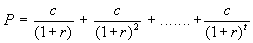Define the discount factor: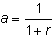Substituting a into the formula, we getTo simplify the present value formula, we need to simplify the expression in the brackets: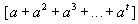To simplify this formula, we first add at+1,at+2, and so on, and then subtract all the terms we added: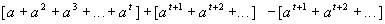We can rewrite this as: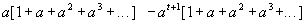Note that the infinite number of terms in each of the brackets is the same. Define:+

Now, observe that V = 1 + aV, which means thatTherefore, the expressionNow, replace a with the discount factor 1/(1 + r) and simplify to get: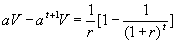We can now simplify the present value formula as follows: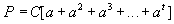Replacing the expression in square brackets with what we derived, we get: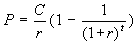which is the annuity formula.

Given the interest rate, r, this formula can be used to compute the present value of the future cash flows. Given the present value, it can be used to compute the interest rate or yield. Finally, given the present value and the interest rate, it can be used to determine the cash flow.

You can use the formula in different ways as you go through Bond Tutor. The topic Time Value of Money in this chapter lets you calculate the present value of each cash flow for an annuity and also lets you see the annuity value through time. In The Term Structure of Interest Rates topic, you can see how the present value is affected by the interest rate and also calculate the interest rate given the present value.